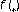We’d like to understand how you use our websites in order to improve them. Register your interest.

# A base-point-free definition of the Lefschetz invariant

## Abstract

In classical Lefschetz-Nielsen theory, one defines the Lefschetz invariantof an endomorphismof a manifold. The definition depends on the fundamental group of, and hence on choosing a base pointand a base path fromto. At times, it is inconvenient or impossible to make these choices. In this paper, we use the fundamental groupoid to define a base-point-free version of the Lefschetz invariant.

## References

1. 1.

Bass H: Euler characteristics and characters of discrete groups. Inventiones Mathematicae 1976,35(1):155–196. 10.1007/BF01390137

2. 2.

Bass H: Traces and Euler characteristics. In Homological Group Theory (Proc. Sympos., Durham, 1977), London Math. Soc. Lecture Note Ser.. Volume 36. Cambridge University Press, Cambridge; 1979:1–26.

3. 3.

Brown RF: The Lefschetz Fixed Point Theorem. Scott, Foresman, Illinois; 1971.

4. 4.

Geoghegan R: Nielsen fixed point theory. In Handbook of Geometric Topology. North-Holland, Amsterdam; 2002:499–521.

5. 5.

Hatcher A: Algebraic Topology. Cambridge University Press, Cambridge; 2002.

6. 6.

Jiang BJ: Lectures on Nielsen Fixed Point Theory, Contemporary Mathematics. Volume 14. American Mathematical Society, Rhode Island; 1983.

7. 7.

Lück W: Transformation Groups and Algebraic K-Theory, Lecture Notes in Mathematics. Volume 1408. Mathematica Gottingensis, Springer, Berlin; 1989.

8. 8.

Lück W: The universal functorial Lefschetz invariant. Fundamenta Mathematicae 1999,161(1–2):167–215.

9. 9.

Stallings J: Centerless groups—an algebraic formulation of Gottlieb's theorem. Topology. An International Journal of Mathematics 1965,4(2):129–134. 10.1016/0040-9383(65)90060-1

## Author information

Authors

### Corresponding author

Correspondence to Vesta Coufal.

## Rights and permissions

Reprints and Permissions

Coufal, V. A base-point-free definition of the Lefschetz invariant. Fixed Point Theory Appl 2006, 34143 (2006). https://doi.org/10.1155/FPTA/2006/34143